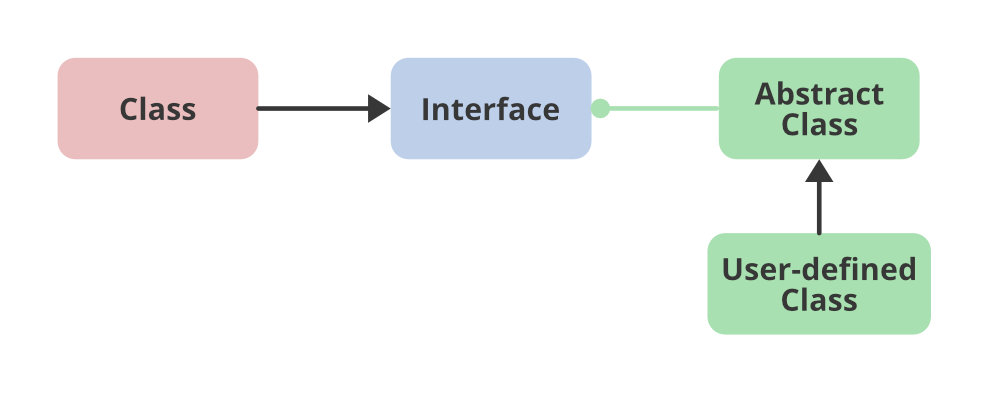# How to Change the Comparator to Return a Descending Order in Java TreeSet?

• Difficulty Level : Basic
• Last Updated : 17 Jan, 2022

Comparator interface is used to order the objects of user-defined classes. A comparator object is capable of comparing two objects of two different classes. TreeSet is one of the most important implementations of the SortedSet interface in Java that uses a Tree for storage. The ordering of the elements is maintained by a set using their natural ordering whether an explicit comparator is provided. This must be consistent with equals if it is to correctly implement the Set interface. It can also be ordered by a Comparator provided at set creation time, depending on which constructor is used.

Syntax: For the comparator is as follows:

`public int compare(Object obj1, Object obj2):`Methods: Getting descending order in TreeSet by changing the comparator.

1. With user-defined class
2. Without user-defined class

Method 1: With user-defined class

To change the comparator, a user-defined class is created that implements the Comparator interface more specifically descendingComparator for which pseudo code is as followed for clear understanding before implementing the same. descendingComparator() is passed as an argument in the TreeSet at the inst stance of creation to get the corresponding descending Order set.

Syntax: Passing descendingComparator()

`Pseudo Code: TreeSet<String> set = new TreeSet<>(new descendingComparator());`
```Pseudo Code: Approach
class descendingComparator implements Comparator<String> {

public int compare(String i1, String i2) {

// compare using compareTo() method
return i2.compareTo(i1);
}
}```

Example

## Java

 `// Java Program to Change the Comparator to Return a``// Descending Order in Java TreeSet` `// Importing all classes of``// java.util package``import` `java.util.*;` `// User0defined class (Helper class)``// New class that implements comparator interface``class` `descendingComparator ``implements` `Comparator {` `    ``public` `int` `compare(Integer i1, Integer i2)``    ``{``        ``// compare using compareTo() method``        ``return` `i2.compareTo(i1);``    ``}``}` `// Main class``public` `class` `GFG {` `    ``// Main driver method``    ``public` `static` `void` `main(String[] args)``    ``{` `        ``// Create a TreeSet and pass descendingComparator()``        ``// as made in another class as parameter``        ``// to it to get Descending Order``        ``TreeSet set``            ``= ``new` `TreeSet<>(``new` `descendingComparator());` `        ``// Adding elements to Treeset``        ``// Custom inputs``        ``set.add(``10``);``        ``set.add(``20``);``        ``set.add(``30``);` `        ``// Print Descending Ordered set in descending order``        ``// as above descendComparator() usage``        ``System.out.println(``"Descending Ordered set : "``                           ``+ set);``    ``}``}`

Method 2: Without user-defined class

Comparator to return a descending order in Java TreeSet at the time of the creation of TreeSet instead of making a new class.

```Pseudo Code: Approach

TreeSet<Integer> set = new TreeSet<Integer>(new Comparator<Integer>()
{
public int compare(Integer i1,Integer i2)
{
// comparing using compareTo() method
return i2.compareTo(i1);
}
});```

Example

## Java

 `// Java Program to Change the Comparator``// to Return Descending Order in Java TreeSet` `// Importing all classes of``// java.util package``import` `java.util.*;` `// Main class``public` `class` `GFG {` `    ``// Main driver method``    ``public` `static` `void` `main(String[] args)``    ``{``        ``// implements comparator interface``        ``// to get Descending Order` `        ``// Method 2: At the time of creation of TreeSet` `        ``// Creating(defining) a TreeSet``        ``TreeSet set = ``new` `TreeSet(``            ``new` `Comparator() {``                ` `              ``// Changing the comparator``                ``public` `int` `compare(Integer i1, Integer i2)``                ``{``                    ``return` `i2.compareTo(i1);``                ``}``            ``});` `        ``// Add elements to above created TreeSet``        ``// Custom inputs``        ``set.add(``10``);``        ``set.add(``20``);``        ``set.add(``30``);` `        ``// Print Descending Ordered TreeSet``        ``System.out.println(``"Descending Ordered Treeset : "``                           ``+ set);``    ``}``}`

Output

`Descending Ordered set : [30, 20, 10]`

My Personal Notes arrow_drop_up